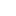TCE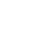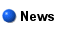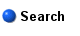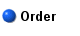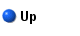## The Chemical Educator

ISSN: 1430-4171 (electronic version)

Abstract Volume 8 Issue 2 (2003) pp 116-121

## The Boltzmann Distribution and Pascal's Triangle

Erich Friedman and W. Tandy Grubbs‡,*

Department of Math and Computer Science and Department of Chemistry, Stetson University, DeLand, FL 32720, wgrubbs@stetson.edu
Received October 17, 2002. Accepted December 10, 2002

Published online: 3 February 2003

Abstract. While Ludwig Boltzmann's contributions to theoretical science are many, the Boltzmann distribution formula is arguably his most important contribution to the field of chemistry. The formula predicts the energy distribution of molecules in an equilibrium system at a given temperature. This distribution in turn governs numerous chemical phenomena, including the intensities of spectral lines, the rates of chemical reactions, and the sedimentation rates of macromolecules, just to name a few. The Boltzmann distribution is normally introduced to students during the statistical mechanics portion of the physical chemistry curriculum. The typical textbook derivation involves applying Lagrange's method of undetermined multipliers to a large system of particles. Undergraduate students, whose mathematics background is often limited to elementary differential and integral calculus, often have trouble grasping this approach because it involves the application of calculus to a multivariable statistical mechanical system. Offered here is an alternate derivation of the Boltzmann distribution formula that instead draws upon some intriguing connections to number theory. The derivation is rigorous and is also pedagogically attractive because one can refer to various graphical energy representations that illustrate how many different ways the particles can be arranged to satisfy the constraints of the system.

Key Words:  In the Classroom; physical chemistry; statistical mechanics; theoretical chemistry

(*) Corresponding author. (E-mail: wgrubbs@stetson.edu)

Article in PDF format (366 KB) HTML format

Issue date: April 1, 2003

© The Chemical Educator 1996-2019